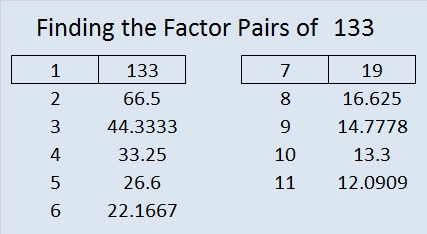# 133 and Level 6

133 is a composite number. 133 = 1 x 133 or 7 x 19. Factors of 133: 1, 7, 19, 133. Prime factorization 133 = 7 x 19.• The divisibility trick for 7 tells us to split 133 into 13 and 3. We double 3 and find the difference between the double and 13. We get 13 (2 x 3)  = 13 – 6  = 7 (an obvious multiple of 7) so 7 is a factor of 133.

133 is never a clue in the FIND THE FACTORS puzzles.Excel file of puzzles and previous week’s solutions: 10 Factors 2014-05-26